# The MPC and MPS

• Intro

• When investment spending increases, there will be an increase in the income and the value of aggregate output by the same amount

• An increase in aggregate output leads to an increase in disposable income and to more consumer spending, which leads to increased output

• How large is the total effect on aggregate output if we sum up all the rounds of spending increases

• It depends on what economists called the marginal propensity to consume (MPC) or the marginal propensity to save (MPS)

• $\text{MPS} = \dfrac{\text{Change in savings}}{\text{Change in income}}$

• $\text{MPC} = \dfrac{\text{Change in consumption}}{\text{Change in income}}$

• MPC + MPS = 1

• The marginal Propensity to Consume

• The MPC is a number between 0 and 1

• If consumers save all their money, the number would be 0

• If consumers spend all their money, the number would be 1

• Usually, the number is between 0 and 1 with industrialized countries having a higher number and developing countries with lower numbers

• If the MPC is 0.8, what's the impact on the total aggregate spending if there's an increase of 50 million in spending?

• Total Increase = Spending Multiplier * Initial Increase = 1/(1-0.8) * 50 = 250

# The Multiplier Effect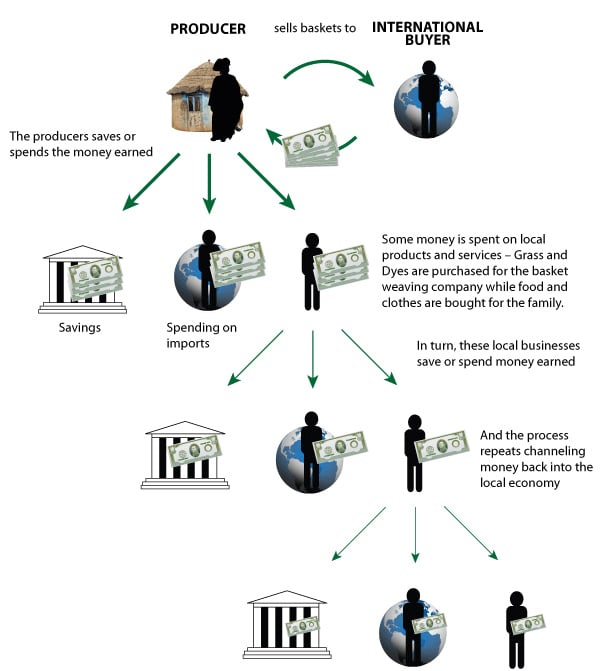• Autonomous change in aggregate spending

• an initial rise or fall in aggregate spending that is the cause, not the result, of a series of income and spending changes
• Multiplier

• ratio of the total change in real GDP caused by an autonomous change in aggregate spending to the size of that autonomous change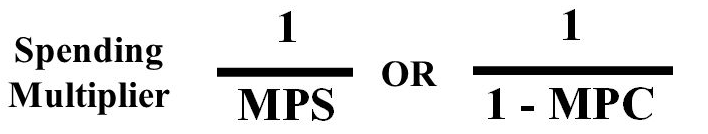• The size of the multiplier depends on the MPC

• The higher the MPC, the more disposable income get recycled back into consumer spending

• The lower the MPC, the more disposable income "leak out" into savings

# Consumption Function

• Consumption function is an equation showing how an individual household's consumer spending changes with disposable income

• Autonomous consumer spending would be the amount spent regardless of income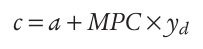• Let's assume that a equals $20,000 and the MPC equals 0.6. What would the consumption be if the income is$100,000? \$200,000?

• c = a + MPC * yd = 20,000 + 0.6 * 100,000 = 80,000

• c = a + MPC * yd = 20,000 + 0.6 * 200,000 = 140,000

• Graph# Shift of the Aggregate Consumption Function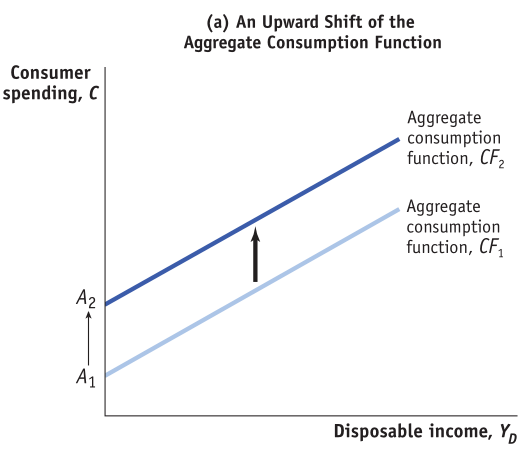• Changes in Expected Future Disposable Income

• If you land a higher-paying job, you will tend to consume more money now even though your current income is the same

• Conversely, if you are worried about a job layoff, you will probably decrease your current expense.

• Changes in Aggregate Wealth

• A booming stock market will tend to increase an individual's wealth, and therefore, his consumption

• A fall in housing prices, conversely, will tend to decrease an individual's net worth, and therefore her consumption

# Investment Spending

• Planned investment spending is the investment spending that businesses intend to undertake during a given time period

• If interest rates goes up, less investment spending occurs.

• If interest rates go down, there is more investment spending

• High expected future growth rate of GDP increases investment

• Low expected future growth rate decreases investment

•• Positive unplanned inventory investment occurs when sales are less than business expects. Excess sales leads to negative unplanned inventory investment

• Rising inventory indicates slowing economy

# Tax (or Government Transfer) Multiplier

• Changes in taxes (or increase in transfer payment) shifts the aggregate demand curve by less than an equal-sized change in government purchases

• The presence of taxed decrease the multiplier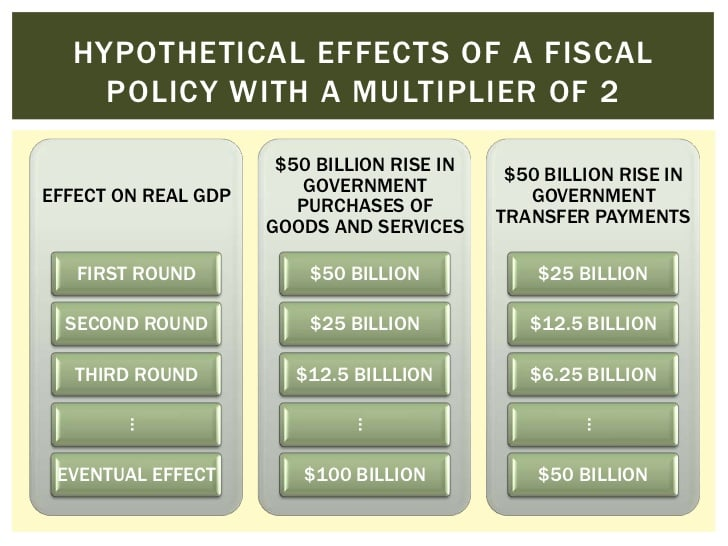# Automatic Stabilizers

• Government spending and taxation rules that cause fiscal policy to be automatically expansionary when the economy contracts and automatically contractionary when the economy expands

• As the economy expands, the multiplier reduces because the increase in income is siphoned off

• As the economy contracts, the multiplier increase because the government is collecting less in taxes (a de facto expansionary policy in the face of a recession)